Next: Non-linear PCA algorithms Up: Algorithms for ICA Previous: Non-linear decorrelation algorithms

## Algorithms for maximum likelihood or infomax estimation

An important class of algorithms consists of those based on maximization of network entropy (infomax) , which is, under some conditions, equivalent to the maximum likelihood approach  (see Section 4.3.1). Usually these algorithms are based on (stochastic) gradient ascent of the objective function. For example, the following algorithm was derived in :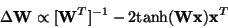(36)

where the tanh function is applied separately on every component of the vector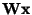, as above. The tanh function is used here because it is the derivative of the log-density of the 'logistic' distribution . This function works for estimation of most super-Gaussian (sparse) independent components; for sub-Gaussian independent components, other functions must be used, see e.g. [124,26,96]. The algorithm in Eq. (36) converges, however, very slowly, as has been noted by several researchers. The convergence may be improved by whitening the data, and especially by using the natural gradient.

The natural (or relative) gradient method simplifies the gradient method considerably, and makes it better conditioned. The principle of the natural gradient [1,2] is based on the geometrical structure of the parameter space, and is related to the principle of relative gradient  that uses the Lie group structure of the ICA problem. In the case of basic ICA, both of these principles amount to multiplying the right-hand side of (36) by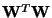. Thus we obtain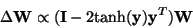(37)

with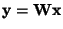. After this modification, the algorithm does not need sphering. Interestingly, this algorithm is a special case of the non-linear decorrelation algorithm in (34), and is closely related to the algorithm in (35).

Finally, in , a Newton method for maximizing the likelihood was introduced. The Newton method converges in fewer iterations, but has the drawback that a matrix inversion (at least approximate) is needed in every iteration.Next: Non-linear PCA algorithms Up: Algorithms for ICA Previous: Non-linear decorrelation algorithms
Aapo Hyvarinen
1999-04-23